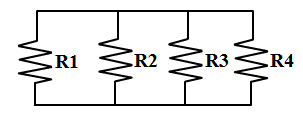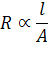Equivalent Resistance of Resistors (Series)

# Our Objective

How to determine the equivalent resistance of two resistors when connected in series.

# The Theory

Before we start learning about series circuits, we need to know what electrical circuits are and what are different types of circuits!

## Electrical Circuit

An electric circuit is simply a closed loop through which charges can continuously move. An electric circuit basically contains a source of electricity, a load resistance, a switch or a key for turning the circuit on or off at one's convenience.

The diagrammatic representation of an electric circuit is called the circuit diagram.

## Types of circuits

When two or more resistors are connected such a way that one end of one resistor is connected to the starting end of the other, then the circuit is called a Series Circuit

Similarly, if the starting ends of two resistors are joined to a point and the terminal ends of the two are combined and given connection to a source of electricity,those circuits are called Parallel Circuit.

Since the current has only one path to take, the current through a series circuit is the same through each resistor.

## Before calculating the equivalent resistance, let me ask you, "What is resistance?"

Voltage can be thought of as the pressure pushing charges along a conductor.

Electrical resistance shows how much energy you need when you move the charges/current through your devices. If you need lots of energy, then the resistance required is also high.

In many materials, the voltage and resistance are connected by Ohm's Law:That is,  the property of a conductor to oppose the flow of charges through it is called its resistance.

It is measured as resistance,All metals are good conductors, so all metal wires have a relatively low resistance.

The longer a piece of wire the higher is its resistance. Double the length means double the resistance.

ie.,The resistance of a piece of wire also depends on its thickness/cross-sectional area A of the conductor. Double the area means half the resistance.  A thin wire has a higher resistance than the same thick piece.

ie.,Finally, resistance of a piece of wire depends on its temperature also. The higher the temperature the higher is the resistance.

## Calculating the effective resistance/total resistance of series circuits:

The total resistance/effective resistance of the circuit is found by simply adding up the resistance values of the   individualresistors. Adding resistors in series always increases the effective resistance.
ie.,  If  Rs  be the resistance of the series combination then,Here, we used Meter Bridge to calculate the effective resistance accurately.

The Meter Bridge is a device for measurement of resistance using the principle of Wheatstone Network.

Let’s do an experiment now ……